American Journal of Theoretical and Applied Statistics
Volume 4, Issue 6, November 2015, Pages: 581-586

The Power of the Pruned Exact Linear Time(PELT) Test in Multiple Changepoint Detection

Gachomo Dorcas Wambui, Gichuhi Anthony Waititu, Anthony Wanjoya

Department of Statistics and Actuarial Science, Jomo Kenyatta University of Agriculture and Technology (JKUAT), Nairobi, Kenya(G. D. Wambui)

Gachomo Dorcas Wambui, Gichuhi Anthony Waititu, Anthony Wanjoya. The Power of the Pruned Exact Linear Time(PELT) Test in Multiple Changepoint Detection.American Journal of Theoretical and Applied Statistics.Vol.4, No. 6, 2015, pp. 581-586. doi: 10.11648/j.ajtas.20150406.30

Abstract: Changepoint detection is the problem of estimating the point at which the statistical properties of a sequence of observations change. Over the years several multiple changepoint search algorithms have been proposed to overcome this challenge. They include binary segmentation algorithm, the Segment neighbourhood algorithm and the Pruned Exact Linear Time (PELT) algorithm. The PELT algorithm is exact and under mild conditions has a computational cost that is linear in the number of data points. PELT is more accurate than binary segmentation and faster as than other exact search methods. However, there is scanty literature on the sensitivity/power of PELT algorithm as the changepoints approach the extremes and as the size of change increases. In this paper, we implemented the PELT algorithm which uses a common approach of detecting changepoints through minimising a cost function over possible numbers and locations of changepoints. The study used simulated data to determine the power of the PELT test. The study investigated the power of the PELT algorithm in relation to the size of the change and the location of changepoints. It was observed that the power of the test, for a given size of change, is almost the same at all changepoints location. Also, the power of the test increases with the increase in size of change.

Keywords: Changepoint, Computational Cost, Detection, Segmentation, Power of the Test

Contents

1. Introduction

Changepoint analysis can be considered to be the identification of points within a data set where the statistical properties change. Detecting such changes is important in many different application areas. Examples include climatology , bioinformatics applications , finance , oceanography  and medical imaging . The challenge in multiple changepoint detection is identifying the optimal number and location of changepoints as the number of solutions increases rapidly with the size of the data. This problem has attracted a lot of attention in statistics, and a variety of search methods have been proposed and implemented .As increasingly longer data sets are being collected, more and more applications require the detection of changes in the distributional properties of such data. As such, it is clearly desirable to use an efficient method for searching the large solution space.

Over the years several multiple changepoint search algorithms have been proposed to overcome this challenge .They include binary segmentation algorithm which from the work of , the Segment neighbourhood algorithm from the work of  and the Pruned Exact Linear Time (PELT) algorithm by . Both segment neighbourhood algorithm and PELT algorithm are exact methods. The PELT algorithm uses a common approach of detecting changepoints through minimization of costs. The computational efficiency of PELT is O(n).To find multiple change points, the PELT algorithm is first applied to the whole data set and iteratively and independently to each partition until no further change points are detected. The main assumption of the PELT algorithm is that the numbers of change points increases linearly with the increase of data set, that is, the change points are spread throughout the data and are not restricted to one portion of the data. The PELT algorithm is exact and under mild conditions has a computational cost that is linear in the number of data points. The method is more accurate compared to approximate search methods and faster compared to other exact search methods.

2. Review of Previous Studies

The changepoint problem has been extensively discussed in literature in recent years. The study of the changepoint problem dates back to  and  which tested the existence of single change point, and  which was motivated by consideration of a "tracking" problem. Gichuhi et al  considered changepoint analysis in a regression setting in case that the responses are dichotomous. They used neural network to detect changepoint and used maximum likelihood estimation method to estimate changepoint when it has been detected.

Multiple changepoint problems also have been considered by many authors including  who tested and estimated linear models with multiple structural changes. Pan and Chen  used modified information criterion to detect multiple changepoints. The problem was also discussed in a Bayesian framework. Yao  considered the problem of estimating a signal which is a step function when one observes the signal plus gaussian noise. Barry and Hartigan  showed that ,with appropriate selection of prior product models,the observations can eventually determine approximately the true partition. Lee  concluded that, under mild assumptions and with respect to a suitable prior distribution, the posterior mode of the number of change points converges to the true number of changepoints in the frequentist sense. The discussion of changepoint problem for dependent observations can be found in  and .

Over the years several multiple changepoint search algorithms have been proposed to overcome this challenge .Binary Segmentation from the work of  is arguably the most established search method used within the changepoint literature. It is an approximate method with computational cost, O (n log n), where n is the number of data points. The segment neighbourhood algorithm by  is an exact test that searches the entire segmentation space using dynamic programming. It has significant computational cost, O(Qn2), where Q is the maximum number of changepoints and n is the number of data points.If the number of changepoints increases linearly as the observed data increases, then Q = O(n) and the computational cost becomes O(n3) . The optimal partitioning method by  improves on the computational efficiency of the segment neighborhood method but cannot match the efficiency of binary segmentation.It can be applied to a slightly smaller class of problems and is an exact approach whose computational cost is O(n2).

PELT method has been used by authors like  who used PELT algorithm in analysing oceanographic time series. They identified the start and end of the storm season automatically. PELT produces quicker and more consistent results than identification `by eye' or assuming that the variability is constant. The analysis focussed on changes in variability within oceanographic data. Madon and Hingrat  used PELT algorithm to analyze animal tracking locations as they are just another kind of time series data. The timing of movement was deciphered by the algorithm for the nine migrant Macqueen’s Bustards and the PELT-TREE method seemed to stand out by its ability to highlight` fine patterns in movement. The segments obtained by the change point analysis highlighted the complexity of the migration strategy of this species making any visual analysis more than subjective.

3. Methodology

3.1. Multiple Changepoint Detection

One commonly used approach to identify multiple changepoints is to minimize: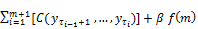(1)

Where C is a cost function for a segment and β f (m) is a penalty to guard against over fitting.

Twice the negative log likelihood is a commonly used cost function in the changepoint literature, although other cost functions such as quadratic loss and cumulative sums are also used or those based on both the segment log-likelihood and the length of the segment. In practice, the most common choice of penalty is one which is linear in the number of changepoints, that is β f (m) = β m. Examples of such penalties include Akaike Information Criterion (AIC) (β= 2p) and Schwarz Information Criterion (SIC, also known as BIC) (β = p log n), where p is the number of additional parameters introduced by adding a changepoint. The PELT method is designed for such linear cost functions

3.2. The Pruned Exact Linear Time (PELT) Method

It is based on the algorithm of , but involves a pruning step within the dynamic program. Jackson, et al. proposed a search method that aims to minimize: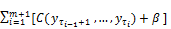(2)

Where C is a cost function for the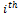segment and β is a penalty to guard against over fitting. Equation 2 is equivalent to equation 1 where f (m) = m.

The PELT method modifies the optimal partitioning method of  by pruning. It combines optimal partitioning and pruning to achieve exact and efficient computational cost which is linear in n. The optimal segmentation is F (n) where,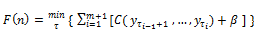(3)

Conditioning on the last point of change,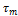and calculating the optimal segmentation of the data up to that changepoint gives,(4)

This could equally be repeated for the second to last, third to last, … changepoints. The recursive nature of this conditioning becomes clearer as one notes that the inner minimisation is reminiscent of equation 3. In fact the inner minimisation is equal to F (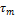) and as such equation 3 can be re-written as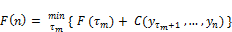(5)

We start by calculating F (1) and then recursively calculate F (2), … , F(n). At each step we store the optimal segmentation up to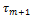. When we reach F (n) the optimal segmentation for the entire data has been identified and the number and location of changepoints have been recorded.

At each step the minimisation overcovers all previous values e.g. when calculating F(3) the minimisation covers= 0, 1, 2. The computational efficiency of the PELT method is achieved by removing candidate values offrom the minimisation at each step. The essence of pruning in this context is to remove those values ofwhich can never be minima from the minimisation performed at each iteration.

3.3. Power of the Test

The changepoint hypothesis problem will be stated as:

H0: No changepoint in the data.

H1: There is changepoint in the data.

The likelihood ratio statistic is: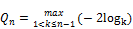(6)

where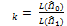, is the ratio of the likelihoods of the sample after and before the change. k is the changepoint and is not fixed and its location is unknown. Q n is an increasing function of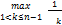and therefore H0 is rejected if,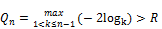(7)

where R is some bound that depends on level of significance and the size n of the sample.

R grows asymptotically as n for a given x depending on the size of the test so that,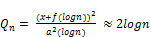(8)

If there is change, then it occurs at a certain point in the data. Thus for a changepoint k,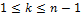and as n, then we have that k,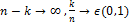. Therefore, this test is consistent, since for a given size α the power of the test converges to 1.

The study will find out the power of the PELT algorithm for finite sample size for specific alternatives of one changepoint. The test rejects the null hypothesis if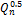> R, where R is the asymptotic critical value which depends on the size of α and the sample size n. For a given level α, the power of the test is the probability of accepting this alternative correctly, that is,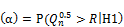(9)

Since the distribution ofunder H1 is not known, simulations will be used to estimate the power of the test. For a sample size n, 1000 replicates will be made and in each replicatewill be estimated. The power function for a given level α will be estimated as,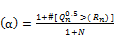(10)

wheredenotes the number of times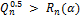.

For a given sample, the power of the test at each changepoint location was be evaluated. For a sample of size n=200, the location of the changepoint k was placed at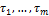= 20, 40, 60, 80, 100, 120, 140, 160, 180. 1000 simulations will be done at each changepoint location. The value of the test statisticin each of 1000 simulations will be computed. Using the critical values R1 and R2 generated using theorem 2.1 or 3.1 in , the power of the test was computed using equation 10. The study analyzed the sensitivity of the test as the changepoints approach the extremes.

1000 simulations were then carried out on the sample to investigate the power of the test in relation to the size of the change Δ. The size of change will be placed at an interval of 0.5. The data was assumed to follow normal distribution.

4. Empirical Results

The study used simulated data.The data was assumed to follow normal distribution with a constant variance. The study analysed the sensitivity of the test as the changepoints approach the extremes. For a given sample, the power of the test at each changepoint location was evaluated. For a sample of size n=200, the location of the changepoint k was then put at n/10, n/5, 3n/10, 2n/5, n/2, 3n/5, 7n/10, 4n/5. This means that k = 20, 40, 60, 80, 100, 120, 140, 160, 180. Using the size of change (Δ = 0.5, 2, 3, 6), 1000 simulations were done at each changepoint location and the power of the test was computed. The results are presented in table 1. A plot of the power of test against the location of changepoint at α = 0.05 is presented in figure 1. Results in table 1 and figure 1show that the power of the PELT method at a given size of change is the almost the same at all changepoint locations.

The study analysed the sensitivity of the test as the size of change increases. For a sample of size n=200, 1000 simulations were done to determine the power of the test at different changes in size of the mean. The size of change denoted by Δ was put at the interval 0.5 .The study considered the following changes in size: 0.5, 1, 1.5, 2, 2.5, 3, 3.5, 4, 4.5, 5, 5.5, 6. The results are presented in table 2. A plot of the power of test against the size of change at α = 0.05 for k=20, k=60 andk=100 is presented in figure 2. Result in table 2 and figure 2 show that the power of the test increases as the size of change increases.

Simulations were also carried out to find out the power of the test as n increases. The simulated data consisted of 5 scenarios with varying lengths, n = c (100,200,300,400,500). The location of the changepoint was put at k = n/2. Using the size of change as 6, 1000 simulations were done using each sample size and the power of the test was computed. The results are presented in table 3. A plot of the 95% average power function at different sample sizes is presented in figure 3.

Table 1. 95% average power function of the PELT test for n= 200.

 power function (α) Size of change Changepoint location 20 40 60 80 100 120 140 160 180 0.5 0 0 0 0 0 0 0 0 0 1.5 0 0.46867 0.46834 0.46914 0.46801 0.46887 0.46766 0.46903 0 2 0.63729 0.63712 0.63701 0.63567 0.63685 0.63659 0.6389 0.63564 0.633 3 0.85354 0.85408 0.85371 0.85349 0.85574 0.85646 0.85594 0.85309 0.85085 6 0.99654 0.99699 0.99679 0.99653 0.99642 0.99652 0.99645 0.99653 0.99622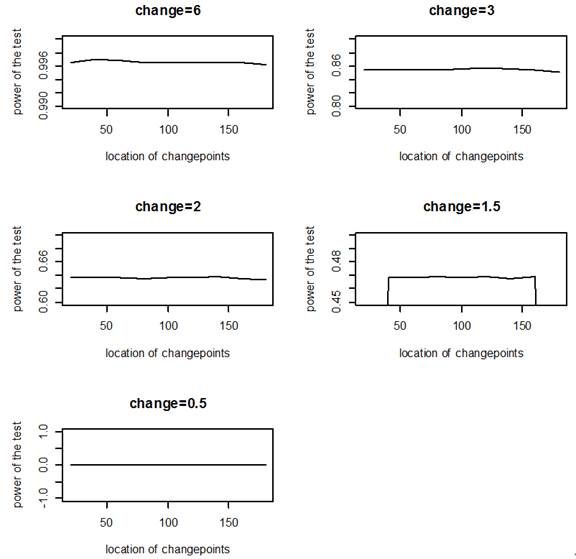Figure 1. The 95% average power function for n=200.

Table 2. 95% average power function for the PELT test at different sizes of change.

 Power function (α) Δ Changepoints location(k) 20 40 60 80 100 120 140 160 180 0.5 0 0 0 0 0 0 0 0 0 1 0 0 0 0 0.278 0 0 0 0 1.5 0 0.46867 0.46834 0.46914 0.46801 0.46887 0.46766 0.46903 0 2 0.63729 0.63712 0.63701 0.63567 0.63685 0.63659 0.6389 0.63564 0.633 2.5 0.76417 0.76441 0.76544 0.76497 0.76609 0.76441 0.76685 0.76362 0.76108 3 0.85354 0.85408 0.85371 0.85349 0.85574 0.85646 0.85594 0.85309 0.85085 3.5 0.91198 0.9133 0.91428 0.91341 0.91613 0.91541 0.91411 0.91324 0.9119 4 0.95154 0.95143 0.95263 0.95083 0.95247 0.95216 0.95173 0.95065 0.95094 4.5 0.97372 0.97542 0.97356 0.97402 0.97484 0.97413 0.97398 0.97387 0.97317 5 0.98676 0.98694 0.98673 0.98717 0.98598 0.98709 0.98655 0.98695 0.98579 5.5 0.99362 0.99298 0.9936 0.99309 0.9936 0.99343 0.9929 0.99331 0.99278 6 0.99654 0.99699 0.99679 0.99653 0.99642 0.99652 0.99645 0.99653 0.99622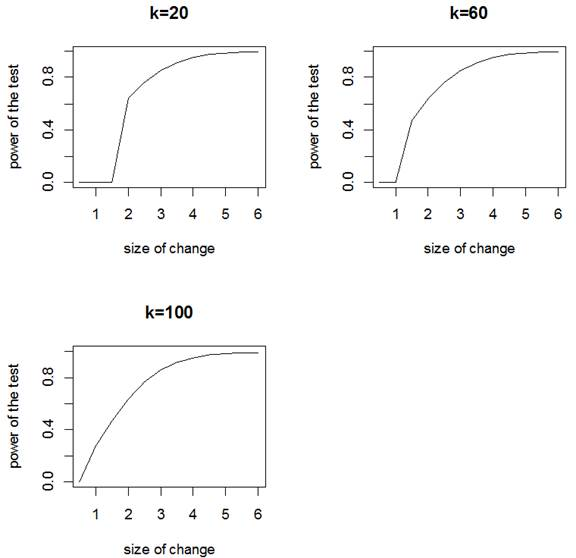Figure 2. % average power function for k=20,k=60 and k=100.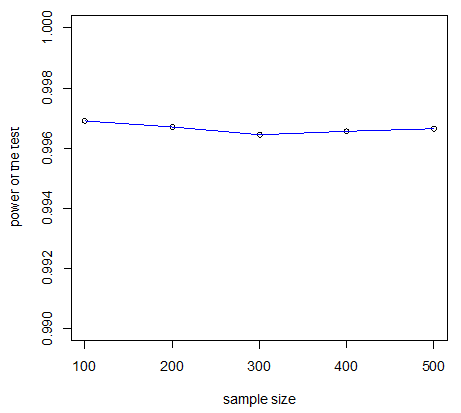Figure 3. The 95% average power function for different sample sizes when changepoint is at n/2.

Table 3. Average power function of the PELT test when changepoint is at n/2 as n increases.

 (α) α Sample size 100 200 300 400 500 0.1 0.99475 0.99436 0.99347 0.99325 0.99311 0.05 0.99691 0.9967 0.99646 0.99657 0.99664 0.01 0.9974 0.99727 0.99695 0.99738 0.9972

5. Conclusion

The study found out that the power of the PELT method increases with increase in the size of change. This means the bigger the change, the more likely it can be detected. It also found out that the power of the test at a given size of change is almost the same at all location of changepoints. This means that the search method is highly sensitive than other methods that are more sensitive at n/2. This is true because PELT method is an exact search method. Its efficient computational cost makes it a good test. Also, the power of the test for different sample sizes is almost the same when the changepoint is placed at k=n/2.This study found the PELT method to be a search method. Therefore, we recommend the use of PELT method by other researchers in other application areas.

References

1. Reeves Jaxk, Chen Jien, Wang, Xiaolan L., lund Robert & Lu Qiqi (2007).A review of comparison of changepoint detection techniques for climate data. Journal of Applied Meteorology and Climatology , 46, 900-915.
2. Erdman, C., & Emerson, J. W. (2008). A fast bayesian change point analysis for the segmentation of microarray data. Bioinformatics, 24(19), 2143-2148
3. Zeileis, A., Shah, A., & Patnaik, I. (2010).Testing,monitoring and dating structural changes in exchange rate regimes. Computational Statistics & Data Analysis , 54 (6), 1696-1706.
4. Killick, R., Eckley, I. A., Jonathan, P., & Eswans, K. (2010). Detection of changes in the characteristics of oceanographic time-series using statistical change point analysis. Ocean Engineering, 37 (13), 1120-1126.
5. Nam, C., Aston, J., & Johansen, A. M. (2012). Quantifying the uncertainity in change points. Journal of Timeseries Analysis, 33(5), 807-823.
6. Scott, A. J., & Knott, M. (1974). Acluster analysis method for grouping means in the analysis of variance. Biometrics , 30, 507-512.
7. Auger Iran E., & Lawrence,Charles E. (1989).Algorithms for the optimal identification of segment neighborhoods. Bulletin of Mathematical Biology, 51, 39-54.
8. Killick Rebecca, Fearnhead P, Eckley Idris A(2012). Optimal detection of change points with a linear computational costs. JASA, 107, 1590-1598
9. Page, E. S. (1954). Continous inspection shemes. Biometrica, 41, 100-116.
10. Page, E. S. (1955). A test for change in a parameter occuring at an unknown point. Biometrica, 42, 523-526.
11. Chernoff, H., & Zacks, S. (1964). Estimating the current mean of normal distribution which is subjected to changes in time. Annals of Mathematical Statistics, 35, 999-1018.
12. Gichuhi Antony Waititu, Franke, Jurgen, & Weizsacker, Heinrich Von (2008).Nonparametric changepoint analysis for bernoulli random variables based on neural networks. KLUEDO.
13. Bai Jushan, & Perron Pierre. (1998). Estimating and testing linear models with multiple structural changes. Econometrica, 66, 47-78.
14. Pan Jianmin, & Chen Jiahua (2006). Application of modified information criterion to multiple change point problems. Journal of Multivariate Analysis , 97, 2221-2241.
15. Yao Yi-Ching (1984). Estimation of a noisy discrete-time step function:Bayes and emperical bayes approaches. The Annals of Statistics , 12, 1434-1447.
16. Barry Daniel, & Hartigan, J. A. (1992). Product partition models for change point problems. The Annals of statistics, 20, 260-279.
17. Lee Chung-Bow (1998). Bayesian estimation of the number of change points. Statistica Sinica, 8, 923-939.
18. Lavielle Marc (1999). Detection of multiple changes in a sequence of dependent variables. Stochastic Processes and their Applications, 83, 79-102.
19. Lai Tze Leung, Liu Haiyan, Xing Haipeng(2005). Autoregressive models with piecewise constant volatility and regression parameters. Statistica Sinica, 15, 279-301.
20. Jackson Brad, Sargle Jeffrey D, Barnes David, Arabhi Sundararajan, Alt Alina, Gioumousis Peter, Gwin Elyus, Sangtrakulcharoen Paungkaew, Tan Linda, Tsai Tun Tao(2005). An algorithm for optimal partitioning of data on an interval. IEEE, Signal Processing Letters, 12(2), 105-108.
21. Killick Rebecca, Eckley Idris A., & Jonathan P. (2011). Efficient Detection of Multiple Changepoints Within An Oceanographic Time Series. In proceedings of the 58th session of ISI. ISI.
22. Madon, Benedicte & Hingrat Yves (2014). Deciphering behavioral changes in animal movement with a "multiple change point algorithm-classification tree" framework. Frontiers in Ecology and Evolution, 2, 1-9.
23. Gombay Edit, & Horvath Lajos (1996). On the Rate of Approximation for Maximum Likelihood tests in Change-point Models. Journal of Multivariate Analysis, 56, 120-152.

 Contents 1. 2. 3. 3.1. 3.2. 3.3. 4. 5.
Article ToolsAbstractPDF(244K)